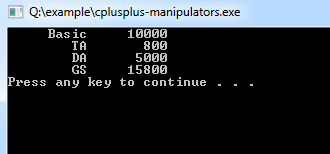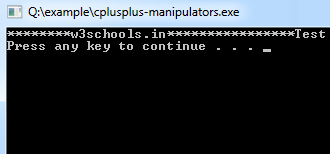## C++ Programming Tutorial Index

In C++, Manipulators are predefined functions that help in formatting output. The output data is manipulated on display according to the programmer's choice. This tutorial will teach you about the various commonly used C++ manipulators.

Here are some commonly used C++ Manipulators:

## `endl` Manipulator

"`endl`" is the line feed operator in C++. It acts as a stream manipulator whose purpose is to feed the entire line and then point the cursor to the beginning of the next line. You can also use "`\n`" (escape sequence) instead of "`endl`" for the same purpose.

## `setw` Manipulator

This Manipulator sets the minimum field width on output.

Syntax:

``setw(x)``

Example:

``````/*
* File: main.cpp
* Author: Gautam
* Created on October 16, 2011, 12:58 PM
*/
#include <iostream>
#include <iomanip>
using namespace std;

int main() {

float basic, ta,da,gs;
basic=10000; ta=800; da=5000;
gs=basic+ta+da;
cout<<setw(10)<<"Basic"<<setw(10)<<basic<<endl
<<setw(10)<<"TA"<<setw(10)<<ta<<endl
<<setw(10)<<"DA"<<setw(10)<<da<<endl
<<setw(10)<<"GS"<<setw(10)<<gs<<endl;
return 0;
}``````

Program Output:## `setfill` Manipulator

The "`setw`" manipulator uses this Manipulator. If a value does not fill a field, then the character specified in the "`setfill`" argument of the Manipulator is used for filling the fields.

Example:

``````/*
* File: main.cpp
* Author: Gautam
* Created on October 16, 2011, 12:58 PM
*/
#include <iostream>
#include <iomanip>
using namespace std;

int main() {
cout << setw(20) << setfill('*') << "w3schools.in" << setw(20) << setfill('*') << "Test" << endl;
}``````

Program Output: